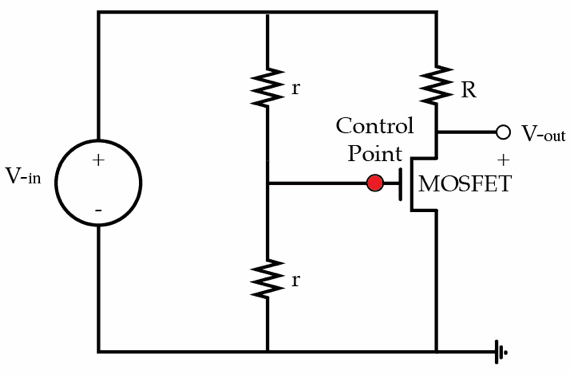# MOSFET

Transistors (marked as MOSFET on the circuit diagram) are electronic devices which act as resistors if the voltage applied to their control point is above the threshold voltage, and as switches (open circuit) if the voltage at the control is below the threshold.

You are given a circuit with a MOSFET with the following characteristics: threshold voltage $V_t = 2 V$, resistance if on $R_{on} = 100 \Omega$. The voltage on the battery is $V_{in} = 10 V$, the dividing resistance is $r = 5 \Omega$ and the control resistance is $R = 50 \Omega$. What is the power in W released on the MOSFET?Details and assumptions

• Hint: You don't have to do extensive calculations to determine the control voltage
×